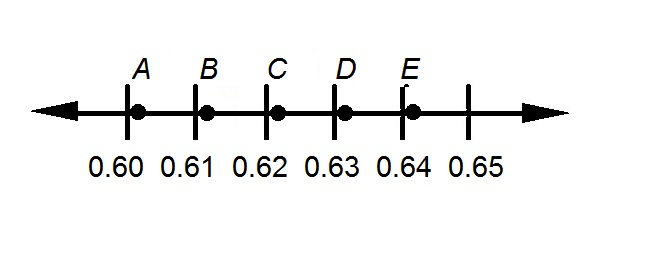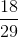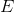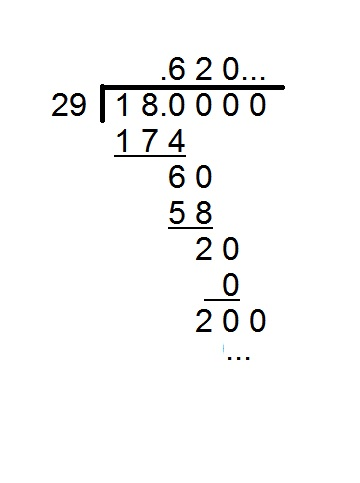## Example Questions

1 3 Next →

### Example Question #21 : How To Find The Decimal Equivalent Of A FractionIn the above number line, which point comes closest to?Divide numerator 18 by denominator 29 to find the decimal equivalent of the fraction:The result is slightly higher than 0.62 - the pointon the number line.